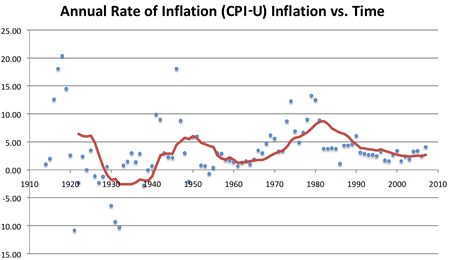How to Calculate the Inflation Rate?

In economic terms, inflation is the sustained increase of level of the goods and services prices. In simple terms, inflation is the increase in prices. By recording the fluctuations of prices of goods and services over the years, it is easy to calculate the rate of inflation.

Calculation of inflation rate using the Consumer Price Index (CPI) is the popular method among most where the Consumer Price Index (CPI) is an indication of the price of goods and services for a consumer. To use this method to calculate the inflation rate you need to know the Consumer Price Index (CPI). However finding the CPI is really easy. You can get this data from the Bureau of Labor Statistics (BLS) as they do survey of consumer prices each monthAnnual Rate of Inflation vs.Time Source: BLS.gov

How to Calculate the Inflation Rate?

To calculate the inflation rate, it is really easy to use an inflation calculator. However, if you want to calculate it manually here is the method.

First you need to determine the base year which you want to calculate inflation rate. Say, if you want to calculate inflation from 2010 to 2016 then 2010 is your base year.

Then you need to find CPI. If you calculate inflation rate for US, then visit the Bureau of Labor Statistics to get this data.

From the CPI data, find the values for base year and current year. Use the values for the below equation and find the inflation rate.

Inflation = (Price Index in Current Year – Price Index in Base Year) * 100

Price Index in Base Year